Friday , March 22 2019
Home / Simple Interest | Interest & Installment# Simple Interest | Interest & Installment

Why someone gives installment paying for a buyer?  It gives some flexibility for the buyer if he gets income on a monthly basis.  Is there any advantage for the seller? Let us see how the installment works!
Suppose, there is a mobile phone for sale at Rs.10,000.  There are two options for the seller. Accepting full money of Rs.10000 in one go, or take some down payment and receiving the remaining amount in “equated monthly/yearly installments”.
If the seller receives the total amount on the purchase, he gets some interest on the sale money.  If he sells it at monthly installment, he makes sure that he sets the installments such that he recovers the interest in several installments.
So the logic works like this:  If the seller sold a product for t months,  Total amount + interest he gets for  t months on sale price = Total installments + interests generated on this installments for the remaining period.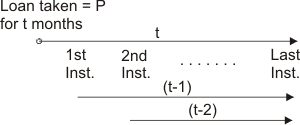For example, if the total loan for 5 months, the seller gets 4 months interest on the 1st installment, 3 months interest on the 2nd installment, 2 months interest on the 3rd installment, 1 month interest on the 4th installment, no interest on 5th installment.
P+P×t×R100×12 = (x+x×(t−1)×R12×100) +(x+x×(t−2)×R12×100) + . . . . . + (x+x×R×212×100) + (x+x×R×112×100) + x

Note: The above formula is for monthly installments.  If installment is asked per year, then no need to divide those terms with 12

General formula for installment calculation: x=P(1+n×r100)n+n(n−1)2×r100
(Note:  If you calculating monthly installments, replace “n” by number of months, and “r” by “r/12”
After simplification of the above formula, you get x=P(100+nr)100n+n(n−1)r2

If Amount will be given (  Principal + interest) then the following formula can be used : 100A100n+n(n−1)r2

Type 1:
If the total loan is taken for 4 months, then situation will be something like this:-
At the end of first month: Interest for next 3 months will be paid
At the end of second month: Interest for next 2 months will be paid
At the end of third month: Interest for next 1 month will be paid
At the end of Forth month: No Interest will be paid
So, if x denotes installment variable, R for rate % per annum, t for time, then every month
Amount to be paid= x + amount of interest
The table will look like this.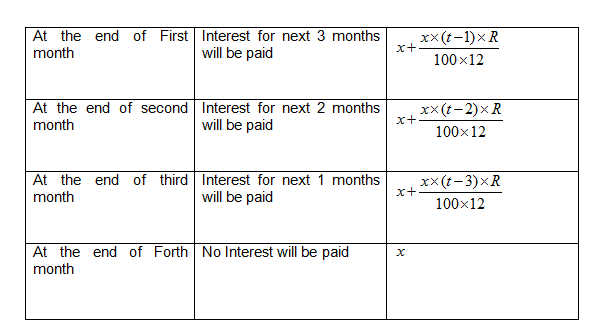This amount will be equal to the principal borrowed and interest given on that for 4 months.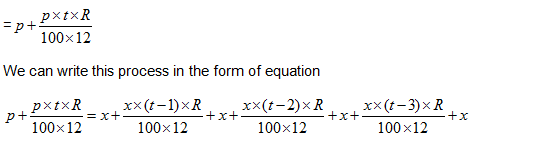Example: A device is available for Rs 5000 cash or Rs 500 down payment followed by 4 equal installments. If the rate of interest charged is 25% per annum simple interest, calculate the monthly installment.

Solution:  After paying a down payment of 500 amount to be paid will be
5000-500=4500
Total amount of installments for 4 months will be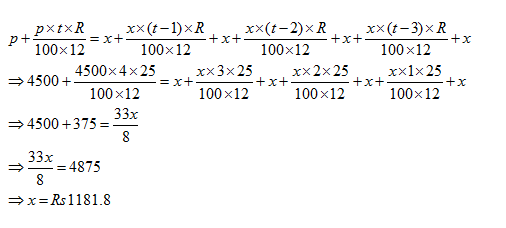Type 2:

Example: What annual installment will discharge a debt of Rs 770 due in 5 years, the rate of interest being 5% per annum?

Solution:Installment paid at the end of 1st ,2nd ,3rd ,4th and 5thyear will be simple interest paid for 4,3,2,1,0years respectively .

Let us suppose the installment given is 100rs
At the end of the first year amount paid will be => 100 + {(5x4x100)/100} = Rs. 120
At the end of the second year amount paid will be =>100 + {(5x3x100)/100} = Rs. 115
At the end of the third year amount paid will be =>100 + {(5x2x100)/100}= Rs 110
At the end of the fourth year amount paid will be =>100 + {(5x1x100)/100}= Rs 105
At the end of the fifth year amount paid will be= Rs 100
Total amount paid is = 120+115+110+105+100= Rs 550
For an amount of Rs 550 annual installment is Rs100
For Rs 770 it will be =(100 x 770)/550 = RS. 140

Shortcut Formula
The annual payment discharged while paying a debt of Rs. A due in n years at the rate of interest r% per annum is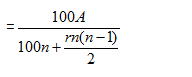Example 2: What annual installment will discharge a loan of Rs. 832 due in 2 yrs @ 8%pa?
Solution:
If Principal = x
x+1.08x= 2.08x=832
x=400
Using the formula, annual installment= [{(100 x 832)/(100×2)+(8x2x1)}] = 400

Type 3:
Example: A mobile worth Rs. 500 can be bought by paying a down payment of Rs.100 and 8 equal installments of Rs. 50 each. Calculate the rate of interest.

Solution:
As it has to be paid in 8 equal installments, the payment on principal to be made will end on the completion of 8 months. He will have to pay (400/8=Rs.50 per month)
Principal for the first month will be 400, for second month=350, for third month=300 and so on.
Total sum or principal=400+350+300+250+200+150+100+50=1800.
Amount= Principal + interest= 400+50=450 (for one month)
Rate = 100* interest/ principal * time
Rate= (100*50)/(1800*1/12)=100/3 %

### Installments Paid With Compound Interest

To calculate the installments paid with compound interest, we use the following formula: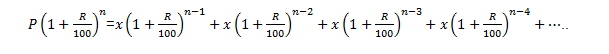Where,
P= Principal
R=rate
n= number of installments
x= Amount of installment

Example: A sum of Rs. 1275 is borrowed at 4% pa compound interest and paid back in 2 equal annual installments. What is the amount of each installment?
Solution:
Let the value of installment be x
Equating the amounts
1275x(1.04)2=x +1.04x
x= Rs. 676

Example: A sum of Rs. 550 is to be repaid in 2 equal annual installments. If rate =20% compounded annually, then the value of each installment will be?
Solution:
Let the value of installment be x
Equating the amounts
550*(1.2)2=x+1.20x
x= Rs. 360

Example: Three equal installments, each of Rs. 300, were paid at the end of the year on a sum borrowed at 20% compounded annually. Find the sum.
Solution:
Rate= 20%
Installment = Rs.300
Principal=?

Putting these values in the formula: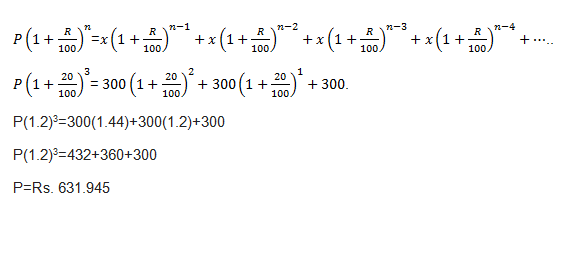Example: A TV set is sold for Rs.880 cash or for Rs.400 cash down payment together with Rs.488 to be paid after one month. Find the rate of interest charged in the installment scheme.
a. Rs. 12%
b. Rs. 10%
c. Rs. 20%
d. Rs. 11%